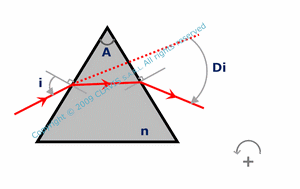and ortutorials

prism in the air or vacuum

A prism is an optical component made of two unparallel plane surfaces. The prism angle A is the angle between these surfaces. In the calculation page, it is assumed that the prism is used in the air or in vacuum. Consequently, the first surface is the interface between air or vacuum (as first medium) and the prism material (as second medium). The second surface is the interface between prism material (as first medium) and air or vacuum (as second medium). n is the refraction index of the prism material.Light transmitted by the prism is deviated by each surface. The total deviation angle Di depends on the prism angle A and the angle of incidence i of the light on the first dioptrum. There is an incidence angle minimizing the total deviation Di. The minimum deviation Dim depends on A and n. As n is larger than air or vacuum refraction index, depending on the angle of incidence of light, total reflexion may occur on the second dioptrum. im is the minimum angle of incidence on the first dioptrum for avoiding total reflexion. im depends on A and n.As the refraction index depends on the wavelength, light rays with different wavelengths have different deviation angles. The dispersion of light deviation dDi between two wavelengths is the difference between the deviation Dis at the smallest wavelength and the deviation Dil at the largest wavelength. dDi depends on A, i, ns and nl, ns and nl being the refraction indexes respectively at the smallest and the largest wavelength.

References

"Optique Fondements et applications" - 2004 - author : José-Philippe Perez.

"Optique géométrique Imagerie et instruments" - 2007 - author : Bernard Balland.

"Optique géométrique paraxiale" - Institut d'optique théorique et appliquée - 1985 - author : Michel Cagnet.

"Formation des images Aberrations" - Institut d'optique théorique et appliquée - 1985 - author : Michel Cagnet.